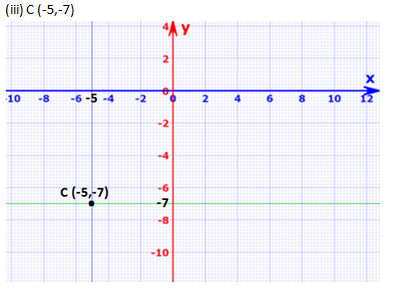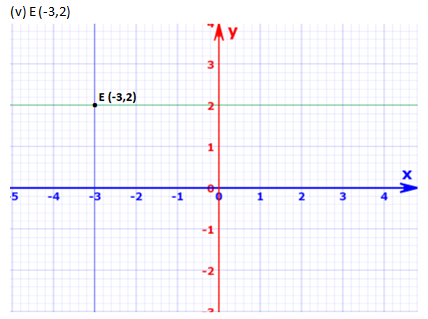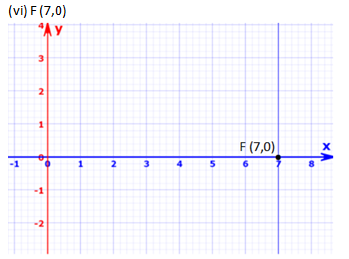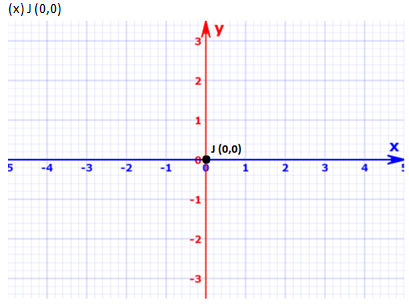# RD Sharma Solutions Class 9 Chapter 11 Coordinate Geometry

Read RD Sharma Solutions Class 9 Chapter 11 Coordinate Geometry below, students should study RD Sharma class 9 Mathematics available on Studiestoday.com with solved questions and answers. These chapter wise answers for class 9 Mathematics have been prepared by teacher of Grade 9. These RD Sharma class 9 Solutions have been designed as per the latest NCERT syllabus for class 9 and if practiced thoroughly can help you to score good marks in standard 9 Mathematics class tests and examinations

Exercise 11.1

Question 1: Plot the following points on the graph paper:
(i) (2,5)
(ii) (4,-3)
(iii) (-5,-7)
(iv) (7,-4)
(v) (-3,2)
(vi) (7,0)
(vii) (-4,0)
(viii) (0,7)
(ix) (0,-4)
(x) (0,0)
Solution:Question 2: Write the coordinates of each of the following points marked in the graph paper.Solution:Hence, the coordinates are A (3,1) B (6,0) C (0,6) D (-3,0) E (-4,3) F (-2,-4) G (0,-5) H (3,-6) P (7,-3) and Q (7,6).